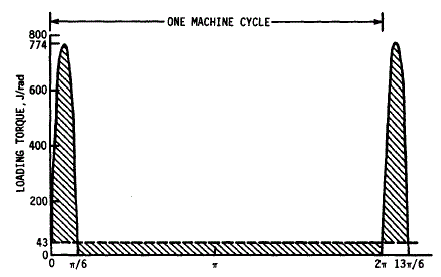Related Resources: mechanics machines

### Flywheel Mass Size Design Equation and Calculator

Flywheel Mass, Size Design Equations and CalculatorThe torque-angle relationship for an engine or machine depends on the the amount of work required. The large variation that is possible between different machine designs shows that dynamic measurement or kinematic analysis is necessary to determine the torque fluctuation. It is often necessary, however, to come up with a rough estimate for preliminary design purposes or for checking the reasonableness of calculated values. For these purposes, the energy variation for a machine or internal-combustion engine can be estimated by:

Energy equation variation flywheel

U = 0.5 J ( ω2max - ω2min )

Polar-mass moment of inertia Fly Wheel. This inertia includes the flywheel inertia and the inertia of all rotating parts, referred to the flywheel speed by multiplying by the square of the ratio of the shaft speeds.

J = U / ( ω2avg Cs )

Coefficient of Speed Variation of a Flywheel

Cs = ( ωmax - ωmin ) / ωavg

Suggested Design Values for the Coefficient of Speed Fluctuation Cs

 Required speed uniformity Cs Very uniform ≤ 0.003 Moderately uniform 0.003-0.012 Some variation acceptable 0.012-0.05 Moderate variation 0.05-0.2 Large variation acceptable ≥ 0.2

The coefficient of energy variation Cu can be approximated for a two-stroke engine with from 1 to 8 cylinders using the equation:

Cu = 7.46 / ( Nc + 1 )3

Coefficient of energy variation for a four-stroke engine with from 1 to 16 cylinders using the two-branched equation:

Cu = 0.8 / ( Nc - 1.4 )1.3 - 0.015

Flywheel Weight:

W = J g / r2a

Cross Sectional Area of Flywheel:

A = J g / ( 2 π ρ r3a )

Power required :

P = T ω

Where:

J = Polar-mass moment of inertia, Ib · s2 · ft (N · s2 · m)
U = Energy Variation, difference between the flywheel energy at maximum speed and at minimum speed, Ib · ft (J)
Nc = Number of cylinders
Cu = Coefficient of energy variation
Cs = Uniformity Speed Constant
K = 33 000 lb · ft · rpm/hp